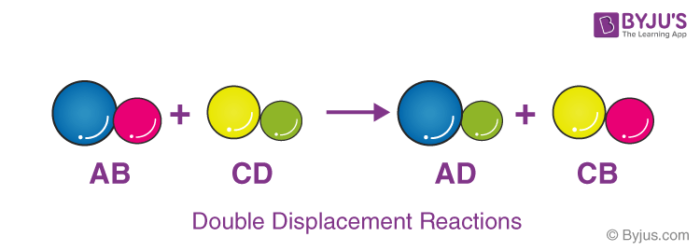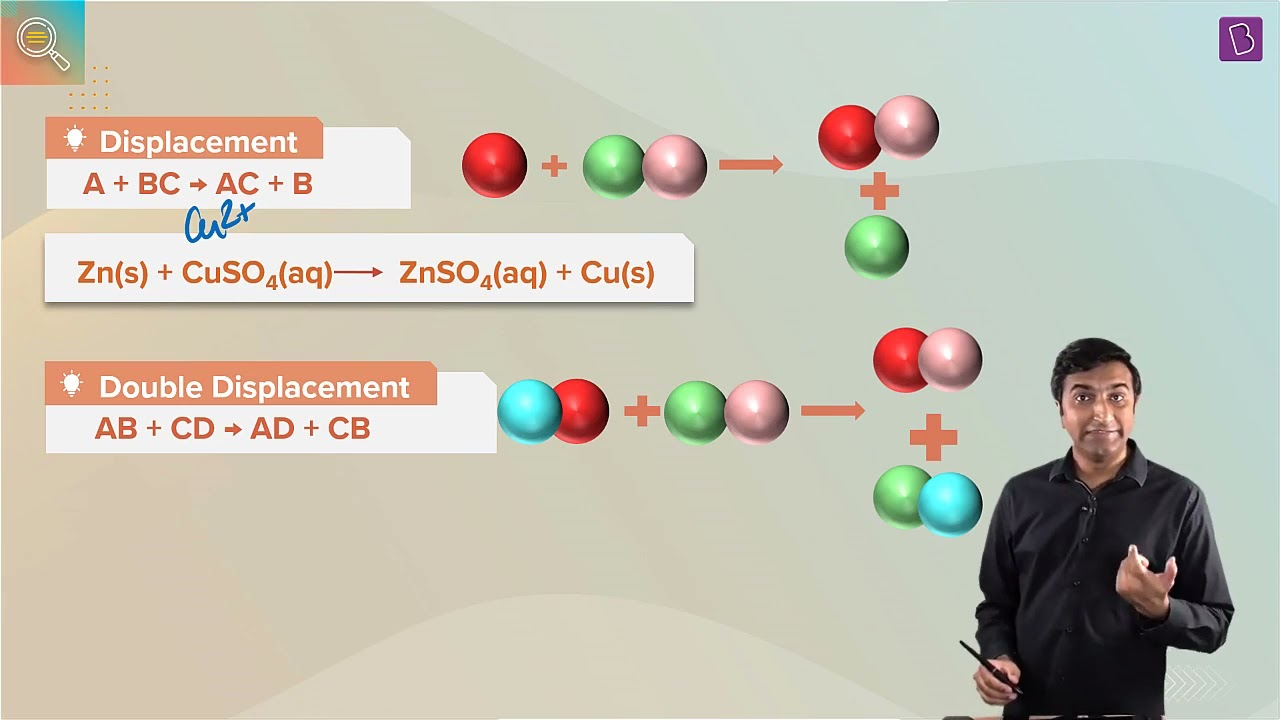# Give examples of double displacement reaction

A displacement reaction is one wherein the atom or a set of atoms is displaced by another atom in a molecule. For instance, when the iron is added to a copper sulphate solution, it displaces the copper metal.

A + B-C → A-C + B

The above equation exists when A is more reactive than B.

A and B have to be either:

• Halogens where C indicates a cation.
• Different metals wherein C indicates an anion

## Double displacement reaction

A double displacement reaction is a type of reaction in which two reactants exchange ions to form two new compounds. Many double displacement reactions occur between ionic compounds that are dissolved in water. A double replacement reaction is represented by the general equation.

##Examples of double displacement reaction

Here are some examples of double displacement reaction:

1. AgNO 3 + NaCl → AgCl + NaNO3
2. 2NaCl + CaSO4 → Na2SO4+ CaCl2
3. H2SO4 + 2LiOH ⇌ Li2SO4 + 2H2O
4. AgNO3 + HCl ⇌ AgCl + HNO3
5. Pb(NO3)2 + 2NaCl ⇌ 2NaNO3+ PbCl2
6. HCl + NaOH → NaCl + H2O
7. Al2(SO4)3 + 6NH4OH → 2Al(OH)3 + 3(NH4)2SO4### Articles to Explore:

4 (112)(287)(43)

#### Choose An Option That Best Describes Your Problem

Thank you. Your Feedback will Help us Serve you better.

#### 1 Comment

1. Vishal Govindrao ingle

Very nice